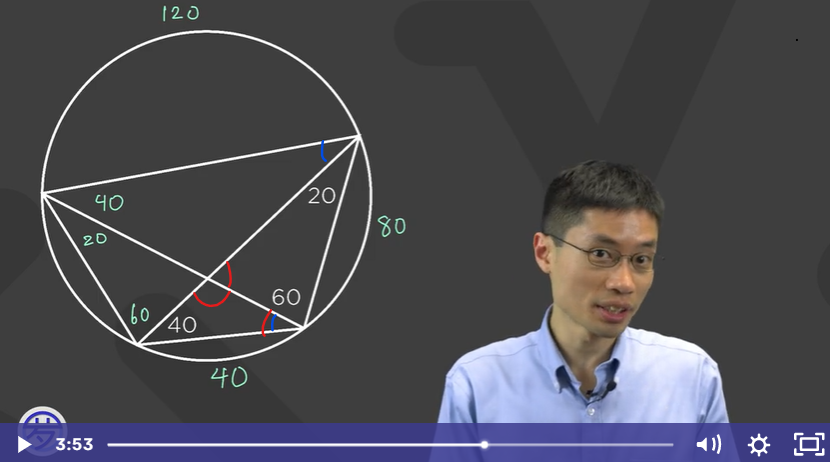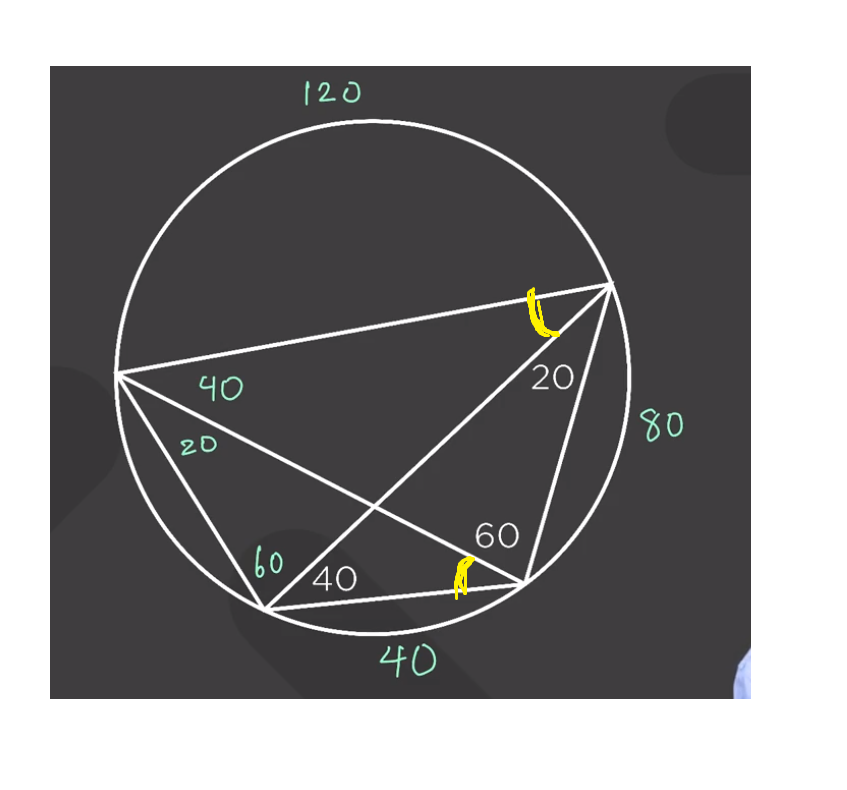# At around 3:53, Prof. Loh said there are two more angles to find, which angles is he talking about? Is it the 60 and the 60 degree angle at the end of the video?

• I don't get why Prof. Loh has to solve these two angles instead of the other ones(blue is what Prof. Loh solved at the end of the video. Red is what the other angles haven't solved). And why did Prof. Loh only talked about the blue angles and not the red ones?• @victorioussheep Good questions! The "two angles that are missing" are these two, marked in yellow, which do turn out to be 60 degrees (I think Prof. Loh also points to them in the video, but I see how it could be a bit unclear).Solving for those yellow angles, rather than the red ones you marked, is a good way to use what you know about inscribed angles and cyclic quadrilaterals. Specifically, Prof. Loh uses the fact that an inscribed angle's measure is half the measure of the arc it cuts off.

But you could definitely solve for the red angles first! Take the triangle with angles 20, 60, and (unknown red angle). The unknown red angle is equal to 100 degrees because the angles of a triangle add to 180. Then, the supplementary red angle is 80 degrees (because 100+80 = 180). Finally, the last red angle equals 180-40-80 = 60 degrees (using the triangle with angle 40, 80, (unknown red/blue angle)), like we found before.

So basically, there are multiple valid ways to solve the problem, and each way emphasizes different methodsfinding the yellow angles first is most useful for illustrating the usefulness of inscribed angles, but you could solve it either way.